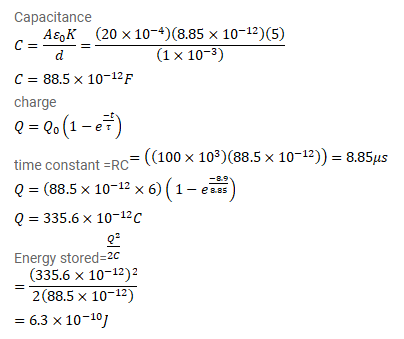# A parallel-plate capacitor has plate area

Question:

A parallel-plate capacitor has plate area $20 \mathrm{~cm}^{2}$, plate separation $1.0 \mathrm{~mm}$ and a dielectric slab of dielectric constant $5.0$ filling up the space between the plates. This capacitor is joined to a battery of emf $6.0 \mathrm{v}$ through a $100 \mathrm{k} \Omega$ resistor. Find the energy of the capacitor $8.9 \mu$ after the connections are made.

Solution: